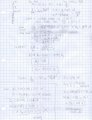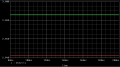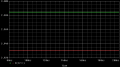# Sedra Smith (5th Ed) Ex D.3.16

#### yitch

Joined Jun 27, 2010
8
The question asks for value of R and junction area.

I tried calculating the current flowing through the diodes using:
v2-v1 = 2.3nVt log (I2/I1)

solving for I2

Since IL = 0,

then the current flowing through the resistor should be I2 which I calculated to be 3.16mA

The voltage across is 15V - 3V = 12V

However the final resistance is 12/3.16mA = 3.79 K ohms

The answer given is 4.8K ohms.

Can someone pls help me understand what I am doing wrong?

And just a quick question, would it be right to equate saturation current Is with the junction area since they are proportional.

Thank you so much for your help#### Georacer

Joined Nov 25, 2009
5,182
I haven't the exact edition of that copy. Could you post the entire exercise?

#### ELECTRONERD

Joined May 26, 2009
1,147
What page are you referring to?

#### yitch

Joined Jun 27, 2010
8
I have taken a photo of the copy, I hope it's clear,

The page is 165

Thank you so much for your help!

#### Ghar

Joined Mar 8, 2010
655
I tried solving it and got a different answer.
It's possible they have a mistake, their problem solutions have mistakes surprisingly often. I don't recall seeing one in an exercise though! Maybe they made some assumptions I didn't, who knows.
Anyway, your approach isn't correct. The diodes do not have 0.7V at 1mA. You are resizing them to satisfy the voltage regulation requirement and rather than provide you with Is you work in terms of that 0.7V 1mA diode.

I scanned my solution...The answer check is a consistency check, it doesn't mean S&S would drop to 2.1 V it just means the values aren't consistent with my equations. We do share an equation though, since R = 1624 / 0.34 = 4.8k

Also just to double check I tried running a SPICE simulation of mine vs his numbers.
This won't be exactly the same but I tried to idealize the model to fit the calculations.
Mine:S&S:Both choices are about the same accuracy initially but mine drops by about 43 mV while S&S drops by 54 mV

#### Georacer

Joined Nov 25, 2009
5,182
I solved it myself too, and found out later that I followed the same path Ghar did, more or less. The only exceptions were that I didn't work with As but straight with Is, wich needed a bit fewer operations. I compared the two Is's at the end to come up with the area ratio. I also calculated the resistance first.
Nontheless, the results were pretty much the same: R=4003 Ohm, A2/A1=0.406, wich can be accounted to third decimal digit precision differences.
The book result are definitely off, same with many other problems. One could check if the book uses the formula:
$$i=I_s \cdot (e^{\frac{v_\tiny{D}}{n \cdot V_\tiny{T}}}-1)$$
although I don't think it will make much difference.

#### Ghar

Joined Mar 8, 2010
655
Yeah that's what I got, it got skewed because I rounded As to 0.4 to calculate resistance.

The book does use that equation but the -1 really wouldn't make a difference. We get the exponential being equal to ~10^12 so 1 doesn't matter at all.

#### yitch

Joined Jun 27, 2010
8

#### aldiger

Joined Jan 12, 2011
1
Thank you very much for solving this exercise.It really help me so much.#### zahidalisiddiqui

Joined Oct 30, 2013
1
Dear,

I solved the question using following technique:

ΔVo/I = 4 x rd => rd = 10Ω.confused: See the solved example 3.7)

Now, as 'rd' is also given by : nVT/Id => Id = VT/rd = 25mV/10Ω = 2.5mA.

R = (15-3)/2.5m = 4.8KΩ.In the solution provided by 'Ghar', I = As x 7.4m, put I = 2.5mA and the problem is solvedAs = 0.34

Best Regards
ζαhiδ

#### WBahn

Joined Mar 31, 2012
26,144
You've just replied to a three-year old thread. Don't expect the OP to be checking back in on it.

We TRY not to revive old threads unless there is a real reason to. But it's very easy to do it by accident (I've done it a few times, too).